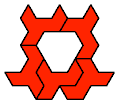# Pairwise Compatibility

## PolyominoesPentomino Compatibility. Given two pentominoes, construct a figure that can be tiled with either.Holeless Pentomino Odd Pairs. Holeless solutions for Livio Zucca's Pentomino Odd Pairs.Pentomino Pair-Single Compatibility. Joint compatibility for two pentominoes with a third.Holeless Hexomino Compatibility. Given two hexominoes, construct a holeless figure that can be tiled with either.Tetromino-Pentomino Compatibility. Given a tetromino and a pentomino, construct a figure that can be tiled with either.Holeless Tetromino-Hexomino Compatibility. Given a tetromino and a hexomino, construct a holeless figure that can be tiled with either.Holeless Pentomino-Hexomino Compatibility. Given a pentomino and a hexomino, construct a holeless figure that can be tiled with either.2×3 Hexomino Compatibility. Which polyominoes are compatible with the 2×3 rectangular hexomino?Holey Heptomino Compatibility. Which polyominoes are compatible with the holey heptomino?H Heptomino Compatibility. Which polyominoes are compatible with the H heptomino?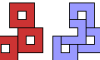Voided Square Octomino Compatibility. Which polyominoes are compatible with the square octomino with a hole in it?Pinwheel Octomino Compatibility. Which polyominoes are compatible with the pinwheel octomino?Square Enneomino Compatibility. Which polyominoes are compatible with the square enneomino?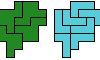A Euphoric Heptomino. This heptominoes is compatible with every polyomino of order 6 or less.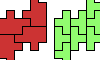Two Euphoric Octominoes. Two octominoes are compatible with every polyomino of order 6 or less.Minimal Incompatibility for Polyominoes. What are the smallest polyominoes that are not compatible with a given polyomino?Addendum to Polypolyominoes. New and smaller polyomino compatibilities for Giovanni Resta's Polypolyominoes.

## Polyiamonds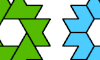Pentiamond Compatibility. Given two pentiamonds, construct a figure that can be tiled with either.Hexiamond Compatibility. Given two hexiamonds, construct a figure that can be tiled with either.Heptiamond Compatibility. Given two heptiamonds, construct a figure that can be tiled with either.Mixed Polyiamond Compatibility. Given two polyiamonds of different orders, construct a figure that can be tiled with either.Triangle Enneiamond Compatibility. Find a figure that can be tiled with a given polyiamond and with the triangular enneiamond.Minimal Incompatibility for Polyiamonds. What are the smallest polyiamonds that are not compatible with a given polyiamond?

## PolyhexesPentahex Compatibility. Given two pentahexes, construct a figure that can be tiled with either.Pentahex Odd Pairs. Given two pentahexes, construct a figure that can be tiled with an odd number of either.Mixed Polyhex Compatibility. Given two polyhexes of different sizes, find a polyhex that can be tiled with either.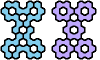Seven Euphoric Pentahexes. Each of these pentahexes is compatible with all 82 hexahexes.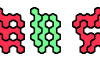Three Euphoric Hexahexes. Each of these hexahexes is compatible with all 82 hexahexes.Ring Hexahex Compatibility. Which polyhexes are compatible with the ring hexahex?Disk Heptahex Compatibility. Which polyhexes are compatible with the disk heptahex?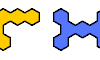Minimal Incompatibility for Polyhexes. What are the smallest polyhexes that are not compatible with a given polyhex?

## Other Plane PolyformsPentapent Compatibility. Figures that can be tiled by two different pentapents.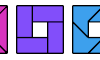Tetrabolo Compatibility. Given two tetraboloes, construct a figure that can be tiled with either.Pentabolo Compatibility. Given two pentaboloes, construct a figure that can be tiled with either.Pentabolo Odd Pairs. Given two pentaboloes, construct a figure that can be tiled with an odd number of either.Diabolo-Tetrabolo Compatibility. Given a diabolo and a tetrabolo, construct a figure that can be tiled with either.Triabolo-Tetrabolo Compatibility. Given a triabolo and a tetrabolo, construct a figure that can be tiled with either.Tetrabolo-Pentabolo Compatibility. Given a tetrabolo and a pentabolo, construct a figure that can be tiled with either.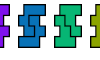Tetrahop Compatibility. Given two tetrahops, construct a figure that can be tiled with either.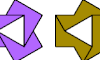Didrafter Compatibility. Given two didrafters, construct a figure that can be tiled with either.Tetrakite Compatibility. Given two tetrakites, construct a figure that can be tiled with either.Trigem Compatibility. Given two trigems, construct a figure that can be tiled with either.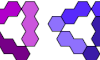Trihing Compatibility. Given two trihings, construct a figure that can be tiled with either.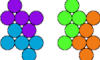Pentapenny Compatibility. Given two pentapennies, construct a figure that can be tiled with either.

## Solid PolyformsTetracube Compatibility. Given two tetracubes, construct a figure that can be tiled with either.Pentacube Compatibility. Given two pentacubes, construct a figure that can be tiled with either.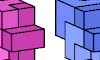Pentacube Odd Pairs. Given two pentacubes, construct a figure that can be tiled with either, using an odd number of each.Flat Hexacube Compatibility. Given two solid hexominoes, construct a figure that can be tiled with either.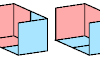Triominoid Compatibility. Given two triominoids, construct a figure that can be tiled with either.

Back to Polyform Compatibility < Polyform Curiosities
Col. George Sicherman [ HOME | MAIL ]